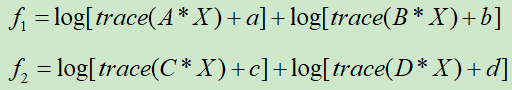# D.C programmingAs showing above， A,B,C,D,X are hermit matrix，the only variable is X. The objective function is (f1-f2), my goal is to maximize (f1-f2), can this problem be solved by D.C programming ? If can , how to deal with it ? Thank you.

`f1 ` is concave, so this term can be handled as is by CVX. `-f2` is convex, so must be “convexified” in the DC algorithm.

See my answers at Can CVX solve the difference of convex functions (D.C.) problems? .

ok, nice ,thank you !